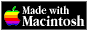THE HYPERCARD CENTER

 HyperTalk Reference
 Home » HyperTalk Reference » Operators and constants Select a destination << Home Stack < HyperTalk Reference Arithmetic operatorsComparison operatorsLogical operatorsString operatorsType and existence operatorsConstants

# Logical operators

### not

Results in` true `if the expression on its right is false, and` false `if the expression on its right is true.

```not (it contains "stack") not (the number of this card is 1) ```

### and

Results in` true `if both the expression to its left and the expression to its right are true.

```(N = 0) and (the number of this card = 1) (the name of this card is "index") and ¬ (the number of this card = 1) and ¬ (myVar is not false) ```

### or

Results in` true `if either the expression to its left or the expression to its right is true.

```(N = 1) or (the number of this card < 10) basicTerm is "button" or ¬ basicTerm is "field" or ¬ basicTerm is "card" or ¬ basicTerm is "background" or ¬ basicTerm is "stack" ```

## Related Topics

Version 0.7b1 (March 24, 2022)Switch to Modern View